﻿ GPS/Galileo实时天顶对流层延迟估计研究文章快速检索 高级检索
 大地测量与地球动力学2019, Vol. 39Issue (7): 728-732  DOI: 10.14075/j.jgg.2019.07.012### 引用本文MAO Yuhui, YUE Zhongqi, XIE Binbin, et al. Research on GPS/Galileo Real-Time Zenith Troposphere Delay Estimation[J]. Journal of Geodesy and Geodynamics, 2019, 39(7): 728-732.### Corresponding author

YUE Zhongqi, assistant engineer, majors in GPS/GNSS precision single point positioning technology, E-mail:490691027@qq.com.

### 第一作者简介

MAO Yuhui, assistant engineer, majors in satellite navigation and positioning technology, E-mail:5241333@qq.com.

### 文章历史

GPS/Galileo实时天顶对流层延迟估计研究

1. 北方自动控制技术研究所，太原市体育路351号，030006

1 PPP观测方程和处理策略

 $\begin{array}{l}{L=\rho+c\left(\mathrm{d} t_{r}-\mathrm{d} t_{s}\right)+T+B+\varepsilon_{L}} \\ {P=\rho+c\left(\mathrm{d} t_{r}-\mathrm{d} t_{s}\right)+T+\varepsilon_{P}}\end{array}$ (1)

 $\begin{array}{c}{T=m f_{\mathrm{h}} Z_{\mathrm{h}}+m f_{\mathrm{w}} Z_{\mathrm{w}}+} \\ {m f_{\mathrm{w}} \cot e \cdot\left(G_{\mathrm{N}} \cos a+G_{\mathrm{E}} \sin a\right)}\end{array}$ (2)

 $\left\{\begin{array}{l}{L^{\mathrm{G}}=\rho^{\mathrm{G}}+c\left(\mathrm{d} t_{r}-\mathrm{d} t_{\mathrm{s}}^{\mathrm{G}}\right)+T^{\mathrm{G}}+B^{\mathrm{G}}+\varepsilon_{L}^{\mathrm{G}}} \\ {L^{\mathrm{E}}=\rho^{\mathrm{E}}+c\left(\mathrm{d} t_{r}-\mathrm{d} t_{s}^{\mathrm{E}}\right)+\mathrm{ISB}_{L}^{\mathrm{E}-\mathrm{G}}+T^{\mathrm{E}}+B^{\mathrm{E}}+\varepsilon_{L}^{\mathrm{E}}}\end{array}\right.$ (3)
 $\left\{\begin{array}{l}{P^{\mathrm{G}}=\rho^{\mathrm{G}}+c\left(\mathrm{d} t_{r}-\mathrm{d} t_{s}^{\mathrm{G}}\right)+T^{\mathrm{G}}+\varepsilon_{P}^{\mathrm{G}}} \\ {P^{\mathrm{E}}=\rho^{\mathrm{E}}+c\left(\mathrm{d} t_{r}-\mathrm{d} t_{s}^{\mathrm{E}}\right)+\mathrm{ISB}_{P}^{\mathrm{E}-\mathrm{G}}+T^{\mathrm{E}}+\varepsilon_{P}^{\mathrm{E}}}\end{array}\right.$ (4)表 1 GPS和Galileo PPP实时ZTD估计策略 Tab. 1 Real-time ZTD estimation strategies of PPP for GPS and Galileo
2 GPS/Galileo数据获取

PPP性能很大程度上取决于轨道和钟差产品的精度。本文所采用的精密预报轨道产品是利用事后精密轨道，通过轨道动力学积分得到。文献利用42~45 h的观测轨道拟合弧段得到更高性能的预报轨道。本文采用45 h的观测拟合轨道弧长，观测拟合轨道选择武汉大学提供的事后精密轨道(ftp://igs.gnsswhu.cn/)；预报轨道采用IGS超快速产品发布的EOP预报值，预报轨道时长为6 h。钟差部分选择武汉大学GNSS中心提供的实时钟差，实时轨道和钟差产品的精度可参照文献。本文基于实时轨道和钟差产品进行事后仿实时解算ZTD。

3 实验结果 3.1 GPS、Galileo、GPS/Galileo解算结果对比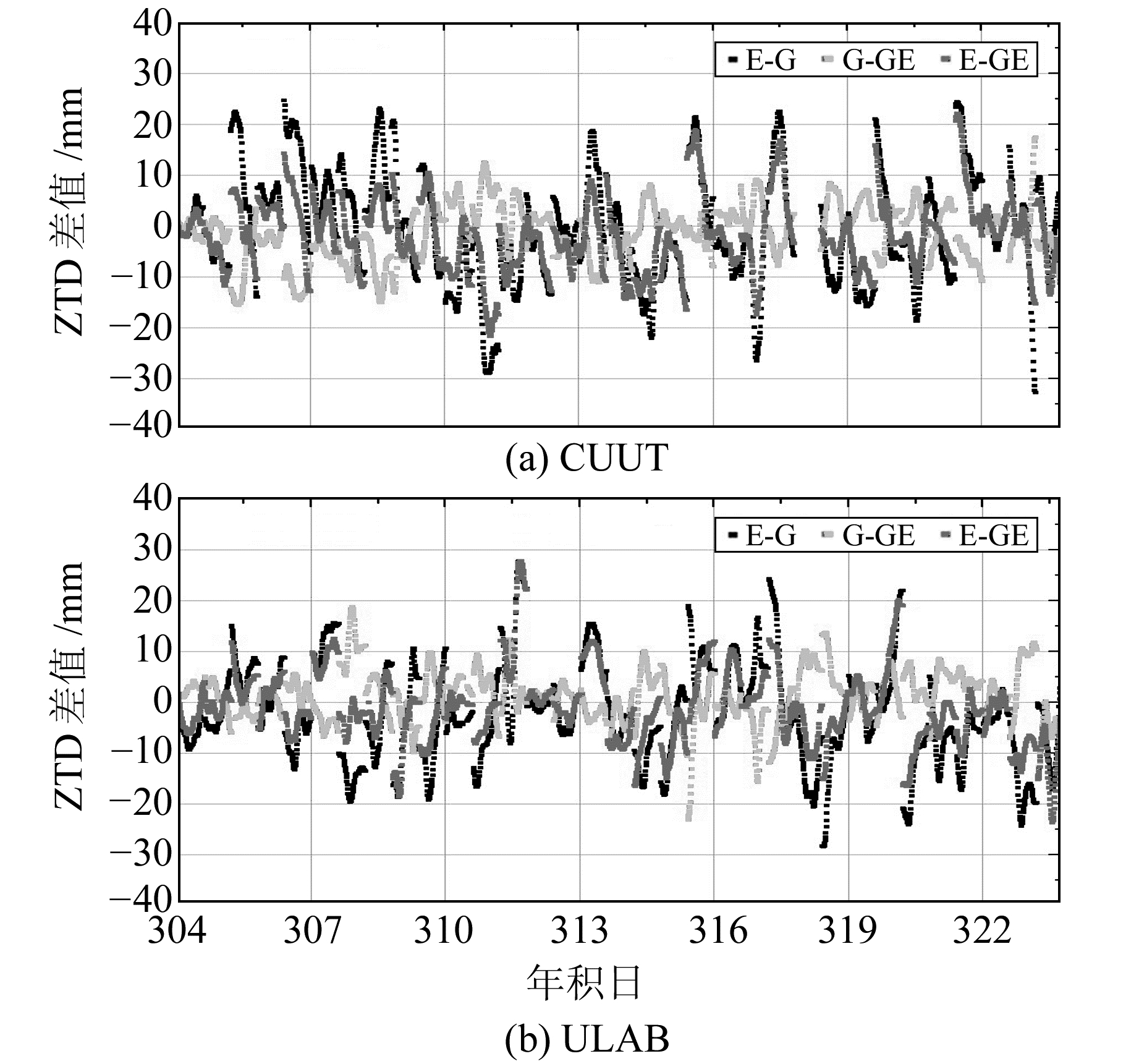图 1 3种解算方法得到的ZTD差值时间序列 Fig. 1 Time series diagrams of ZTD difference obtained by three solutions表 2 各测站3种实时估计方法得到的ZTD间的相关系数统计 Tab. 2 Correlation coefficient of the ZTD obtained by real-time estimation at each measured station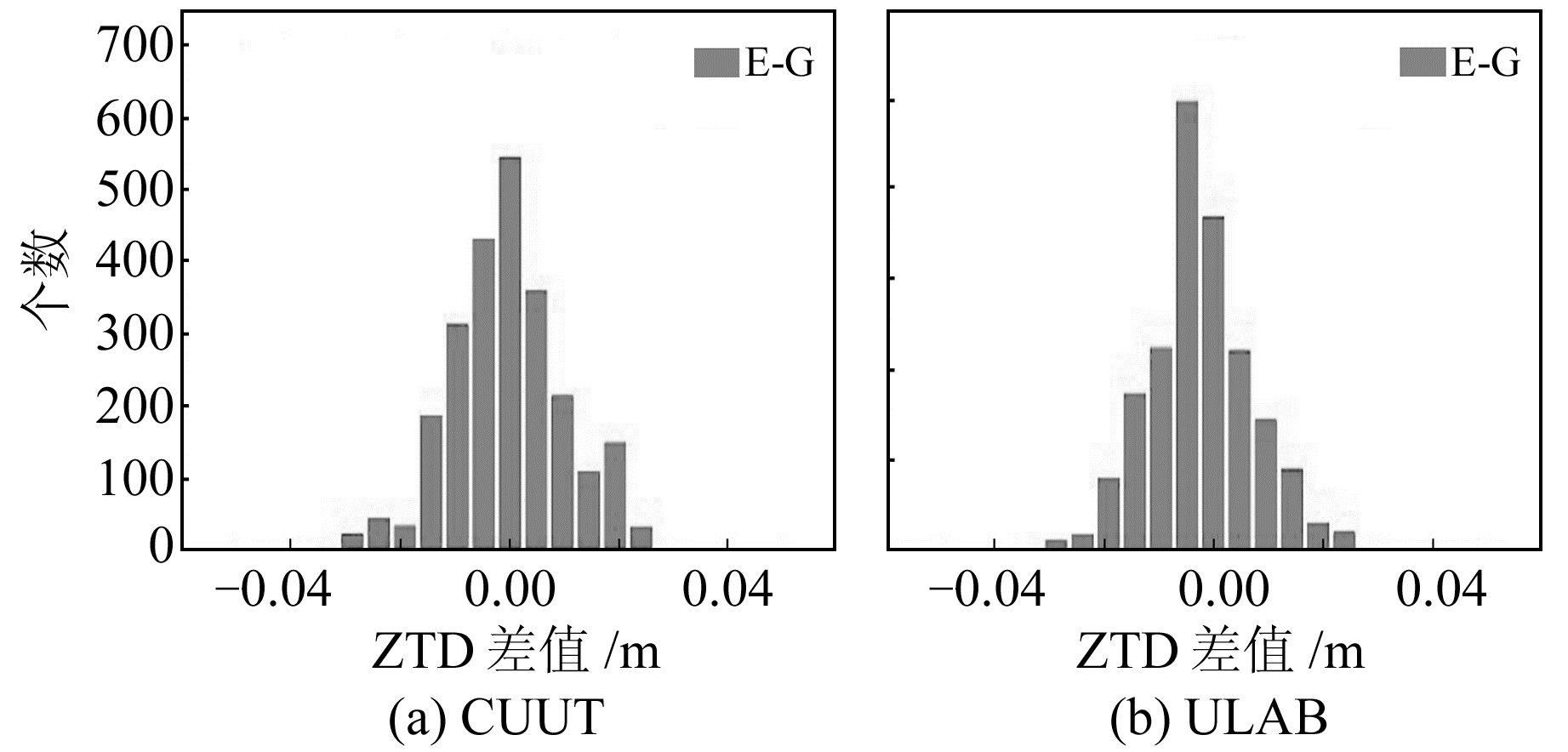图 2 alileo解和GPS解ZTD差值的分布 Fig. 2 ZTD difference distribution of Galileo solution and GPS solution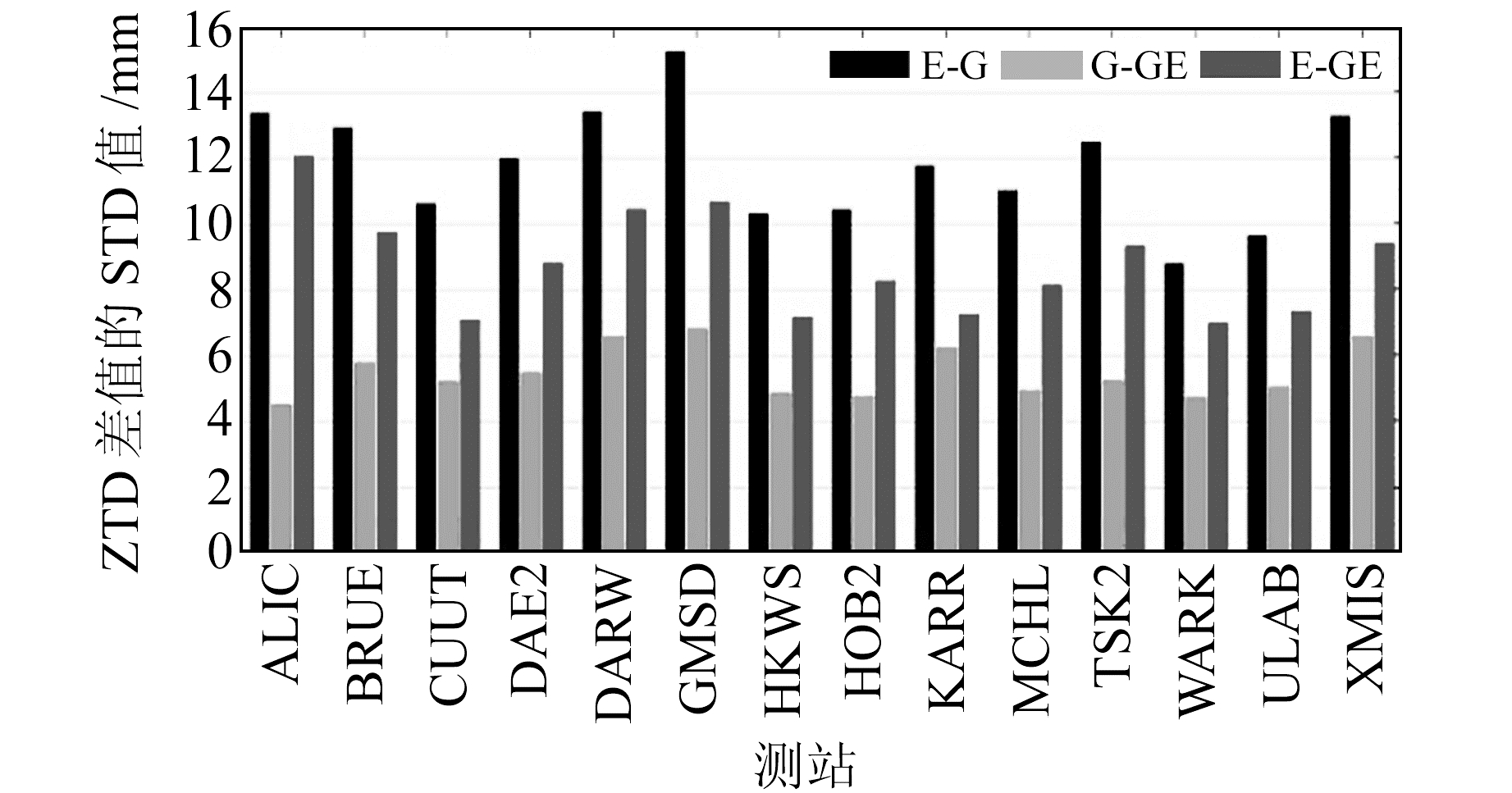图 3 Galileo解和GPS解ZTD差值的STD值 Fig. 3 STD values of ZTD difference of Galileo solution and GPS solution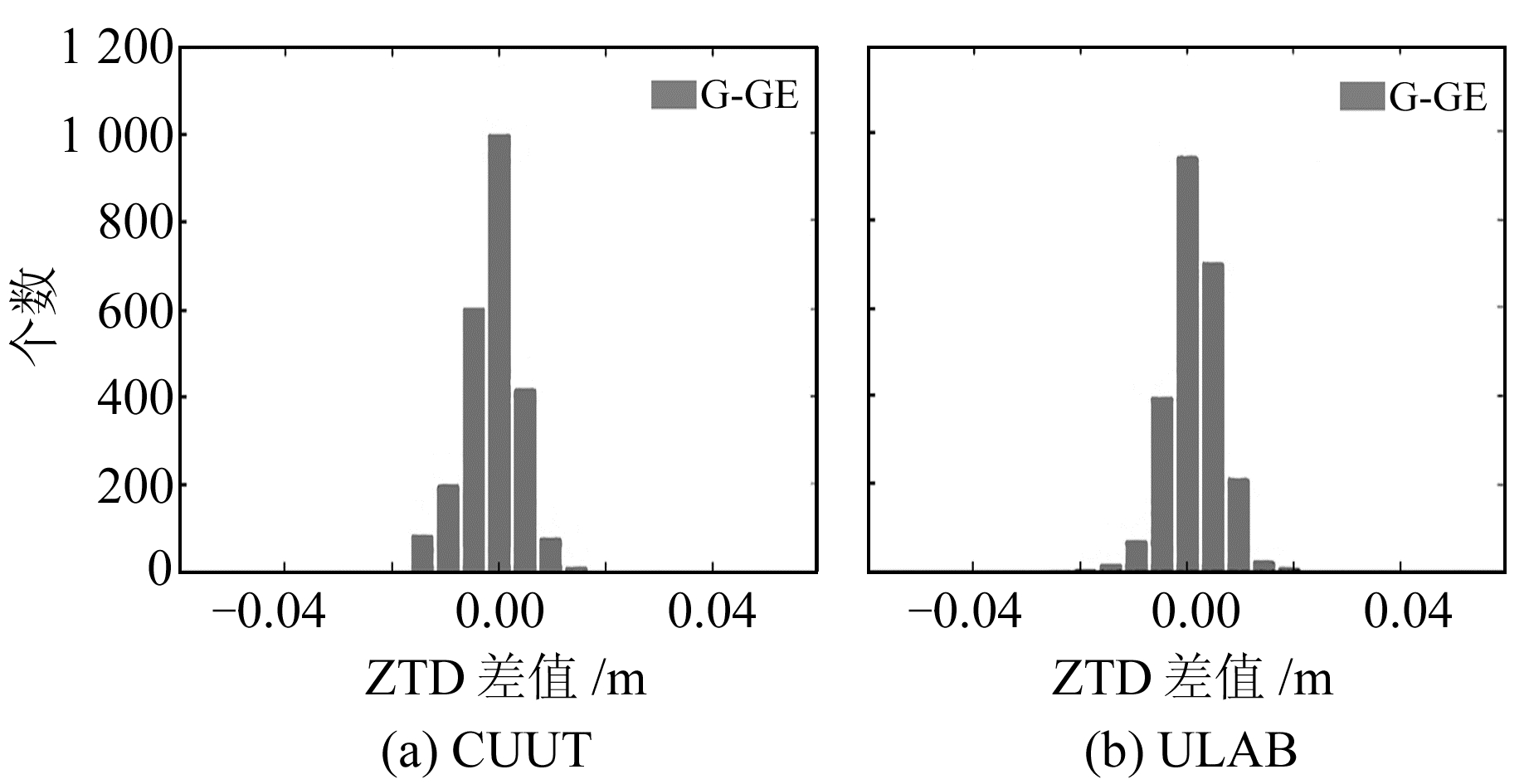图 4 单GPS解和GPS/Galileo组合解ZTD差值的分布 Fig. 4 Distribution of ZTD difference of single GPS solution and GPS/Galileo combination solution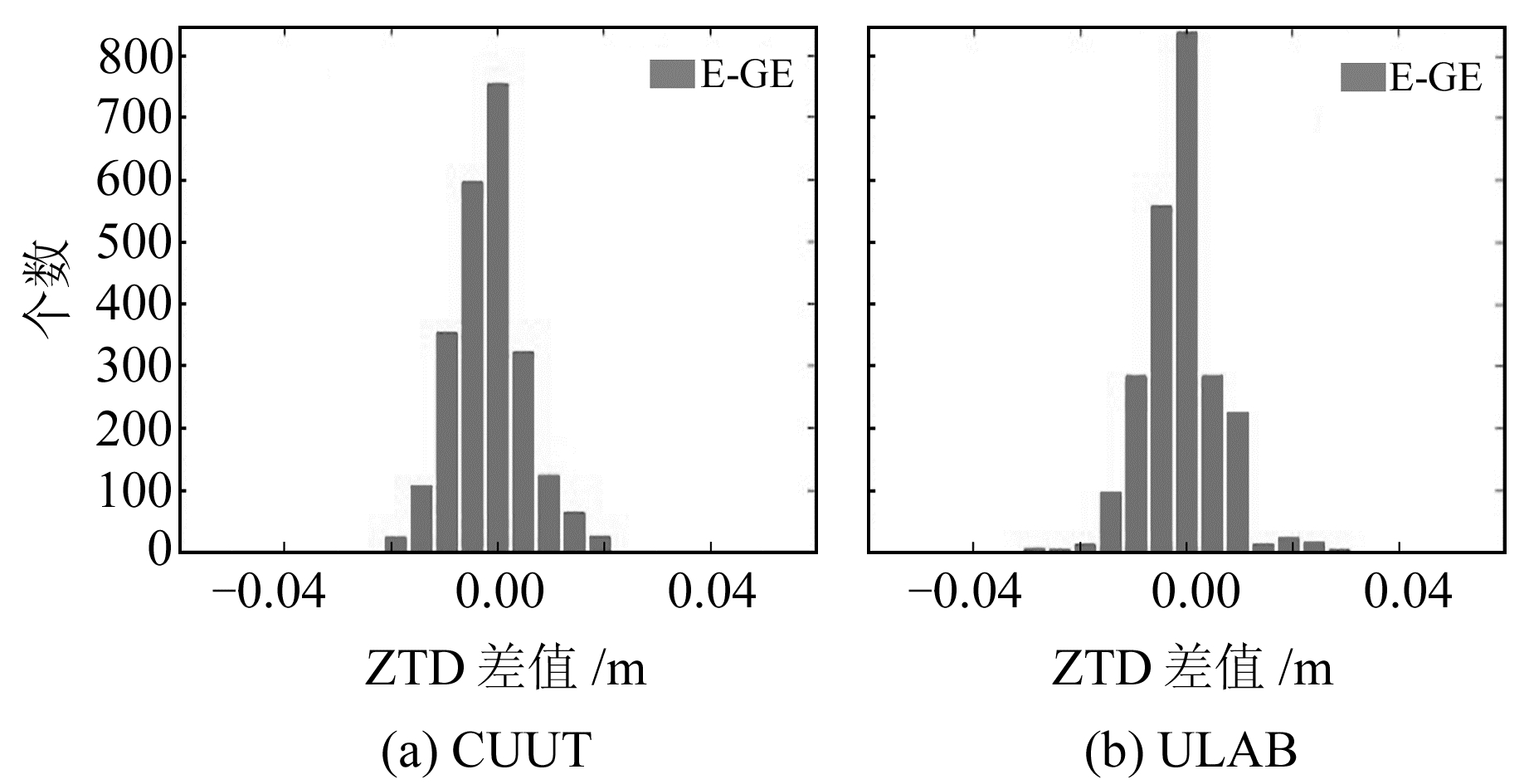图 5 单Galileo解和GPS/Galileo组合解ZTD差值的分布 Fig. 5 Distribution of ZTD difference of single Galileo solution and GPS/Galileo combination solution
3.2 与IGS最终产品对比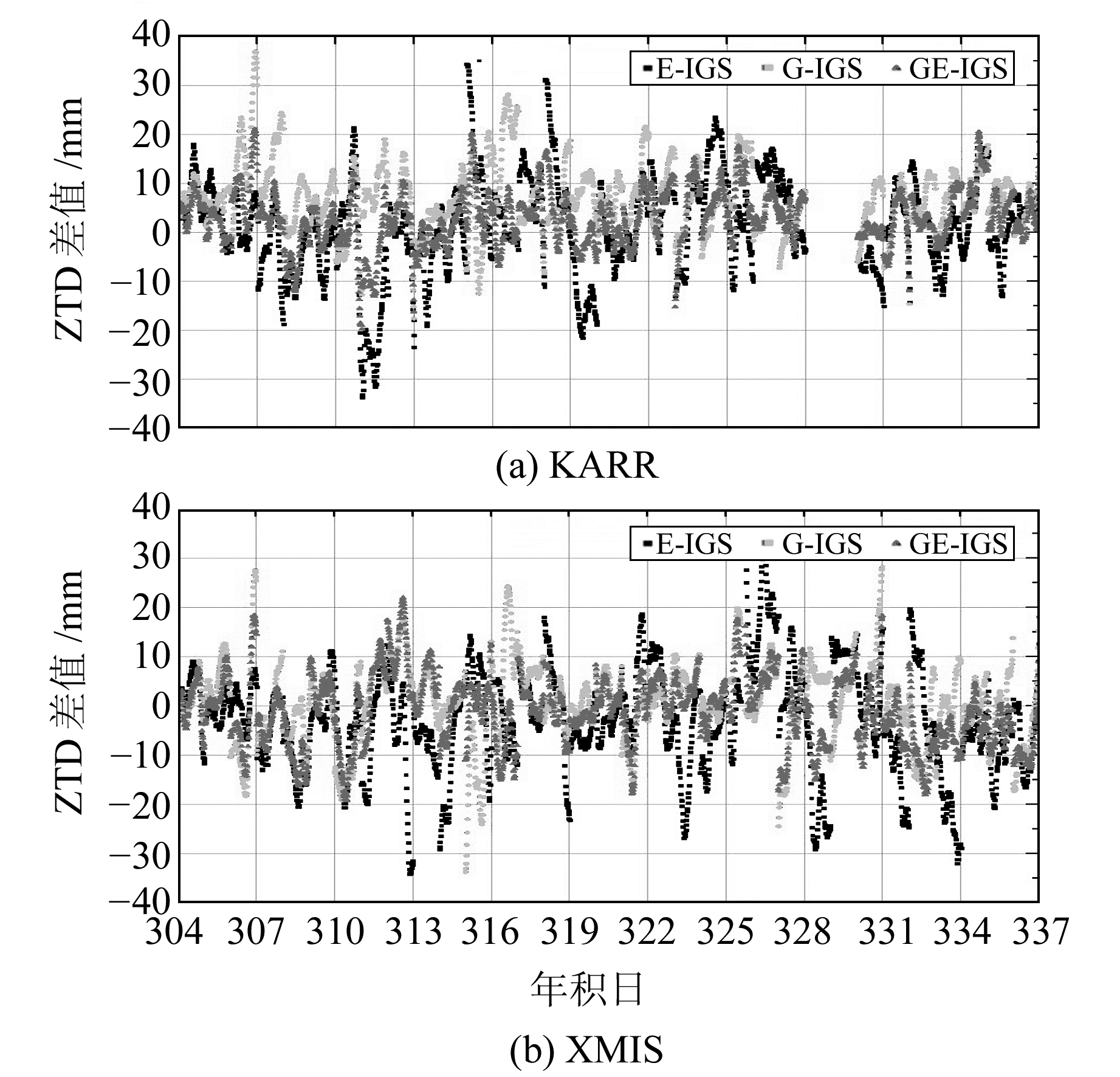图 6 3种解算方法和IGS最终解的ZTD差值时间序列 Fig. 6 ZTD difference time series of IGS final solution and three solutions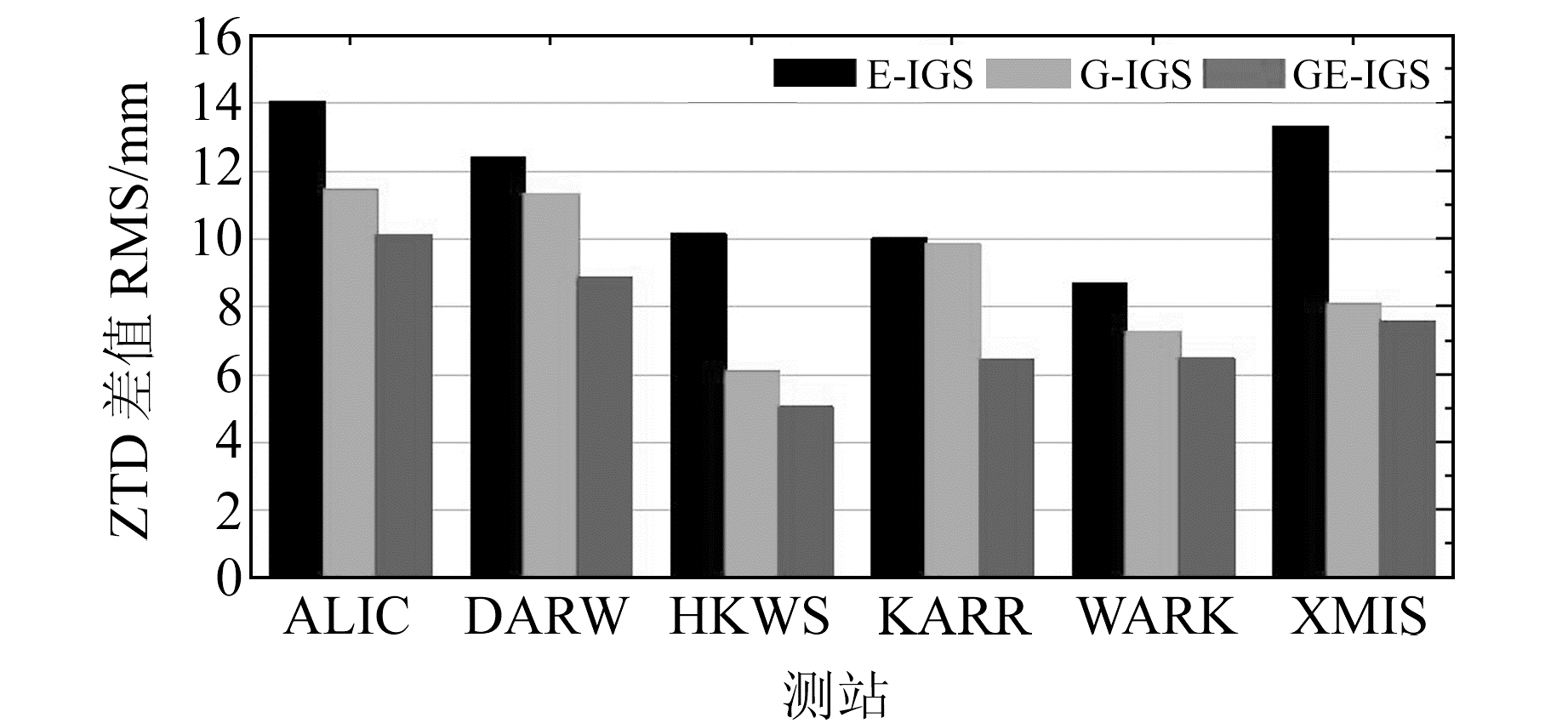图 7 3种解算方法和IGS最终解ZTD差值的RMS Fig. 7 RMS of ZTD difference of IGS final solutions and three solutions
4 结语

  Bevis M, Businger S, Herring T A, et al. GPS Meteorology: Remote Sensing of Atmospheric Water Vapor Using the Global Positioning System[J]. Journal of Geophysical Research Atmospheres, 1992, 97(D14): 15 787-15 801 DOI:10.1029/92JD01517 (0)  Rocken C, van Hove T, Ware R. Near Real-Time Sensing of Atmospheric Water Vapor[J]. Geophys Res Lett, 1997, 24: 3 221-3 224 DOI:10.1029/97GL03312 (0)  Askne J, Nordius H. Estimation of Tropospheric Delay for Microwaves from Surface Weather Data[J]. Radio Science, 2016, 22(3): 379-386 (0)  Davis J L, Herring T A, Shapiro I I, et al. Geodesy by Radio Interferometry: Effects of Atmospheric Modeling Errors on Estimates of Baseline Length[J]. Radio Science, 1985, 20(6): 1 593-1 607 DOI:10.1029/RS020i006p01593 (0)  Zumberge J F, Heflin M B, Jefferson D C, et al. Precise Point Positioning for the Efficient and Robust Analysis of GPS Data from Large Networks[J]. Journal of Geophysical Research Solid Earth, 1997, 102(B3): 5 005-5 017 DOI:10.1029/96JB03860 (0)  Lu C, Li X, Nilsson T, et al. Real-Time Retrieval of Precipitable Water Vapor from GPS and Beidou Observations[J]. Journal of Geodesy, 2015, 89(9): 843-856 DOI:10.1007/s00190-015-0818-0 (0)  Lu C, Li X, Ge M, et al. Estimation and Evaluation of Real-Time Precipitable Water Vapor from GLONASS and GPS[J]. GPS Solutions, 2016, 20(4): 1-11 (0)  Li X, Dick G, Lu C, et al. Multi-GNSS Meteorology: Real-Time Retrieving of Atmospheric Water Vapor From Beidou, Galileo, GLONASS, and GPS Observations[J]. IEEE Transactions on Geoscience & Remote Sensing, 2015, 53(12): 6 385-6 393 (0)  Ding W, Teferle F N, Kazmierski K, et al. An Evaluation of Real-Time Troposphere Estimation Based on GNSS Precise Point Positioning[J]. Journal of Geophysical Research Atmospheres, 2017, 122 (0)  Saastamoinen J. Contributions to the Theory of Atmospheric Refraction. Part Ⅱ. Refraction Corrections in Satellite Geodesy[J]. Bull Geod, 1973, 107(1): 13-34 (0)  Boehm J, Niell A, Tregoning P, et al. Global Mapping Function (GMF): A New Empirical Mapping Function Based on Numerical Weather Model Data[J]. Geophysical Research Letters, 2006, 33(7) (0)  Geng T, Zhang P, Wang W, et al. Comparison of Ultra-Rapid Orbit Prediction Strategies for GPS, GLONASS, Galileo and Beidou[J]. Sensors, 2018, 18(2): 477 DOI:10.3390/s18020477 (0)  Wang Z, Li Z, Wang L, et al. Assessment of Multiple GNSS Real-Time SSR Products from Different Analysis Centers[J]. ISPRS International Journal of Geo-Information, 2018, 7(3): 85 DOI:10.3390/ijgi7030085 (0)
Research on GPS/Galileo Real-Time Zenith Troposphere Delay Estimation
MAO Yuhui1YUE Zhongqi1XIE Binbin1     DUAN Jinjue1     WANG Yintao1
1. North Automatic Control Technology Institute, 531 Tiyu Road, Taiyuan 030006, China
Abstract: This paper calculates ZTD for Galileo, GPS and GPS/Galileo, with PPP ZTD estimation method using observation data from 14 MGEX stations. The RMSs of Galileo-GPS ZTD differences range from 8 mm to 16 mm. The average of RMSs of total stations is 12.3 mm, which proves that Galileo-ZTD agrees well with GPS-ZTD and that the accuracy of Galileo real-time ZTD is satisfactory. Meanwhile, IGS final ZTD products are adopted to assess the performance of each solution. The result shows that the accuracy is improved by 5%-35% when compared to GPS single solutions, and 25%-51% to Galileo single solutions.
Key words: GPS; Galileo; zenith troposphere delay; real-time; PPP